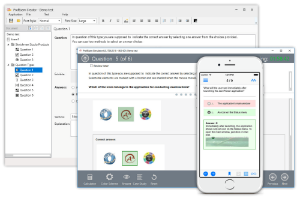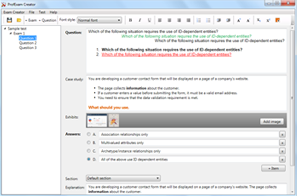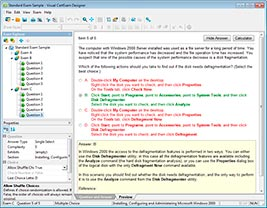## File Info

 Exam Analyzing Big Data with Microsoft R Number 70-773 File Name Unnamed.PracticeTest.70-773.2019-04-10.1e.17q.vcex Size 159 Kb Posted April 10, 2019 Downloads 25

### How to open VCEX & EXAM Files?

Files with VCEX & EXAM extensions can be opened by ProfExam Simulator.

Discount: 20%

## Demo Questions

Question 1
Note: This question is part of a series of questions that present the same scenario. Each question in the series contains a unique solution that might meet the stated goals. Some question sets might have more than one correct solution, while others might not have a correct solution.
After you answer a question in this sections, you will NOT be able to return to it. As a result, these questions will not appear in the review screen.

You use dplyrXdf, and you discover that after you exit the session, the output files that were created were deleted.
You need to prevent the files from being deleted.
Solution: You remove all instances of the file.remove method.
Does this meet the goal?

• A: Yes
• B: No

Question 2
Note: This question is part of a series of questions that present the same scenario. Each question in the series contains a unique solution that might meet the stated goals. Some question sets might have more than one correct solution, while others might not have a correct solution.
After you answer a question in this sections, you will NOT be able to return to it. As a result, these questions will not appear in the review screen.

You have a Microsoft SQL Server instance that has R Services (In-Database) installed.
You need to monitor the R jobs that are sent to SQL Server.
Solution: You register an Extended Events package.
Does this meet the goal?

• A: Yes
• B: No

Question 3
Note: This question is part of a series of questions that use the same or similar answer choices. An answer choice may be correct for more than one question in the series. Each question is independent of the other questions in this series. Information and details provided in a question apply only to that question.

You need to evaluate the significance of coefficients that are produced by using a model that was estimated already.
Which function should you use?

• A: rxPredict
• B: rxLogit
• C: summary
• D: rxLinMod
• E: rxTweedie
• F: stepAic
• G: rxTransform
• H: rxDataStep

Question 4
You plan to read data from an Oracle database table and to store the data in the file system for later processing by dplyrXdf. The size of the data is larger than the memory on the server to be used for modelling.
You need to ensure that the data can be processed by dplyrXdf in the least amount of time possible.
How should you transfer the data from the Oracle database?

• A: Define a data source to the Oracle database server by using RxOdbcData. Use rxImport to save the data to a comma-separated values(CSV) file.
• B: Use the RODBC library, connect to the Oracle database server by using odbcConnect, and then use rxDataStep to export the data to a comma-separated values (CSV) file.
• C: Define a data source to the Oracle database server by using RxOdbcData,and then use rxImport to save the data to an XDF file.
• D: Use the RODBC library, connect to the Oracle database server by using odbcConnect, and then use rxSplit to save the data to multiple comma-separated values (CSV) file.

Question 5
You are running a parallel function that uses the following R code segment. (Line numbers are included for reference only.)
01 cp <- 0.01 xval <- 0 maxdepth <- 5
02
03 (form, data = “segmentationDataBig”, maxDepth = maxdepth, cp = cp, xval = xval, blocksPerRead = 250
You need to complete the R code. The solution must support chunking.
Which function should insert at line 02?

• A: rxBTrees
• B: rxExec
• C: rxDForest
• D: rxDTree

Question 6
You need to build a model that looks at the probability of an outcome. You must regulate between L1 and L2.
Which classification method should you use?

• A: Two-Class Neutral Network
• B: Two-Class Support Vector Machine
• C: Two-Class Decision Forest
• D: Two-Class Logistic Regression

Question 7
You perform an analysis that produces the decision tree shown in the exhibit. (Click the Exhibit button.)How many leaf nodes are there on the tree?

• A: 2
• B: 3
• C: 5
• D: 7

Question 8
You need to run a large data tree model by using rxDForest. The model must use cross validation.
Which rxDForest option should you use?

• A: maxSurrogate
• B: maxNumBins
• C: maxDepth
• D: maxCompete
• E: xVal

Question 9
You have the following regression forest.Which variable contributes the most to the dependent variable?

• A: stack.loss
• B: Water.Temp
• C: Air.Flow
• D: Acid.Conc

Question 10
Note: This question is part of a series of questions that use the same or similar answer choices. An answer choice may be correct for more than one question in the series. Each question is independent of the other questions in this series. Information and details provided in a question apply only to that question.

You have a data source that is larger than memory.
You need to visualize the distribution of the values for a variable in the data source.
What should you use?

• A: the Describe package
• B: the rxHistogram function
• C: the rxSummary function
• D: the rxQuantile function
• E: the rxCube function
• F: the summary function
• G: the rxCrossTabs function
• H: the ggplot2 package

CONNECT US##### PROFEXAM WITH A 20% DISCOUNT

You can buy ProfExam with a 20% discount..

HOW TO OPEN VCEX AND EXAM FILES

Use ProfExam Simulator to open VCEX and EXAM filesHOW TO OPEN VCE FILES

Use VCE Exam Simulator to open VCE files